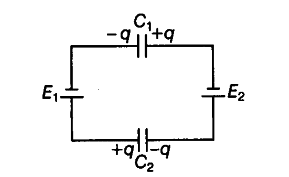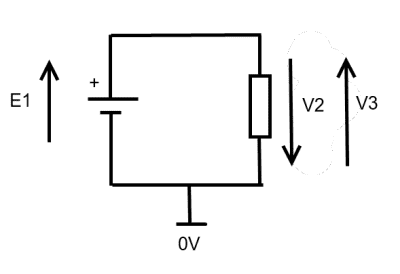# Potential difference across capacitor in a network

Suyash Singh

## Homework Statement

Determine the potential difference across the plates of the capacitor of the network shown in the figure.## Homework Equations

kirchoff's loop rule
q=vc
3. The attempt at a solutio
applying kirchoff's law
starting at bottom right point,
q/c2-E1+q/c1+E2=0
=>E2-E1=-q/c1-q/c2
now i am confused what to do further

V1=-q/c1 and V2=q/c2

phyzguy
You don't need Kirchoff's law. Look at the second of the two relevant equations that you wrote down. Give q and c, what is v?

Suyash Singh
You don't need Kirchoff's law. Look at the second of the two relevant equations that you wrote down. Give q and c, what is v?
v=q/c
but why is v1 negative and v2 positive?

cnh1995
Homework Helper
Gold Member
v=q/c
but why is v1 negative and v2 positive?
Negative or positive with respect to what?
Looks like they are assuming the ground to be at the negative terminal of E2.

CWatters
Homework Helper
Gold Member
+1

I think it is a badly written question. Is that the exact wording?

•SammyS
Suyash Singh
+1

I think it is a badly written question. Is that the exact wording?
yes it was asked in All India CBSE board 2013.

Suyash Singh
Negative or positive with respect to what?
Looks like they are assuming the ground to be at the negative terminal of E2.
the ground is taken at zero potential in our syllabus.

CWatters
Homework Helper
Gold Member
Even if the 0V node was specified the polarity of the voltages isn't defined. Consider this circuit...If E1 = 10V then V3 = 10V and V2 = -10V. See how the polarity depends on the direction of the arrow I drew on the circuit.

So I would argue that in your circuit all of the following are valid answers...

V1=+q/c1 and V2=+q/c2
V1=-q/c1 and V2=+q/c2
V1=+q/c1 and V2=-q/c2
V1=-q/c1, and V2=-q/c2

•cnh1995 and Suyash Singh
Suyash Singh